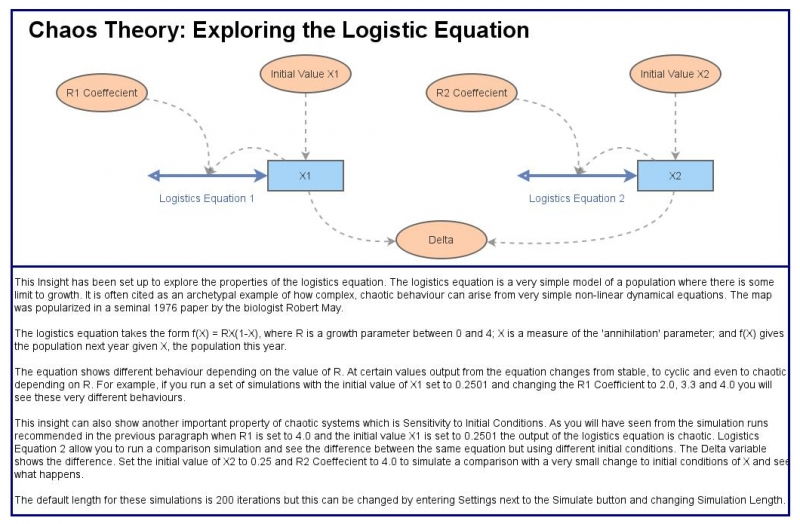Chaos 101: Logistic EquationThis Insight has been set up to explore the properties of the logistics equation.  The logistics equation is a very simple model of a population where there is some limit to growth.  It is often cited as an archetypal example of how complex, chaotic behaviour can arise from very simple non-linear dynamical equations. The map was popularized in a seminal 1976 paper by the biologist Robert May.
You can change the input values of the equations below and see the results by hitting the Simulate button.